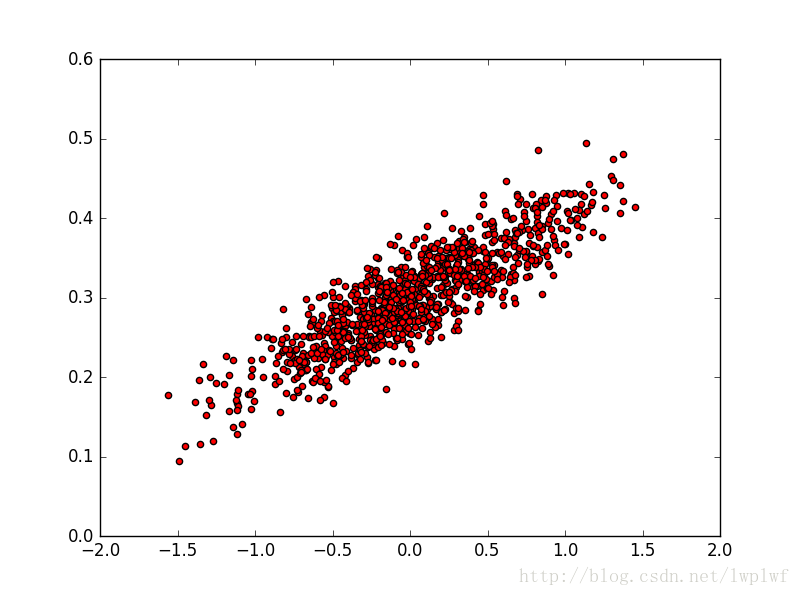﻿ TensorFlow神经网络构造线性回归模型示例教程_python_脚本之家
python# TensorFlow神经网络构造线性回归模型示例教程

```import numpy as np
import tensorflow as tf
import matplotlib.pyplot as plt
# 随机生成1000个点，围绕在y=0.1x+0.3的直线周围
num_points = 1000
vectors_set = []
for i in range(num_points):
x1 = np.random.normal(0.0, 0.55)
# np.random.normal(mean,stdev,size)给出均值为mean，标准差为stdev的高斯随机数（场），当size赋值时，如：size=100，表示返回100个高斯随机数。
y1 = x1 * 0.1 + 0.3 + np.random.normal(0.0, 0.03)
# 后面加的高斯分布为人为噪声
vectors_set.append([x1, y1])
# 生成一些样本
x_data = [v for v in vectors_set]
y_data = [v for v in vectors_set]
plt.scatter(x_data, y_data, c='r')
plt.show()
# 构造1维的w矩阵，取值是随机初始化权重参数为[-1, 1]之间的随机数
w = tf.Variable(tf.random_uniform(, -1.0, 1.0), name='w')
# 构造1维的b矩阵，初始化为0
b = tf.Variable(tf.zeros(), name='b')
# 建立回归公式，经过计算得出估计值y
y = w * x_data +b

# 定义loss函数,估计值y和实际值y_data之间的均方误差作为损失
loss = tf.reduce_mean(tf.square(y - y_data), name='loss')
# 采用梯度下降法来优化参数，学习率为0.5
# train相当于一个优化器，训练的过程就是最小化loss
train = optimizer.minimize(loss, name='train')
sess = tf.Session()
# 全局变量的初始化
init = tf.global_variables_initializer()
sess.run(init)
# 打印初始化的w和b
print('w = ', sess.run(w), 'b = ', sess.run(b), 'loss = ', sess.run(loss))
# 训练迭代20次
for step in range(20):
sess.run(train)
# 打印训练好的w和b
print('w = ', sess.run(w), 'b = ', sess.run(b), 'loss = ', sess.run(loss))
```